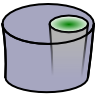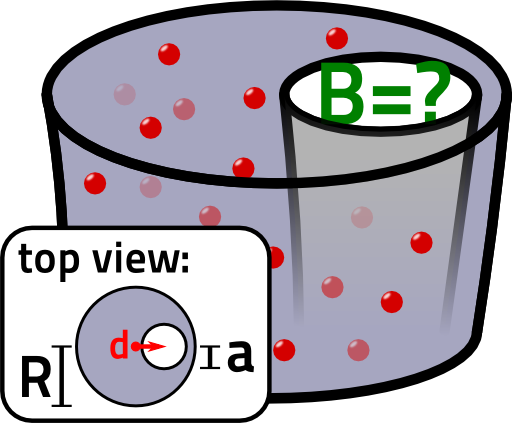## The Magnetic Field of a Hollow WireWhat happens if we take a wire, drill a hole in it and set some current in motion? Will we encounter a magnetic field?

## Problem StatementA wire with radius $$R$$ shall carry a constant current density $$j_{0}$$. Inside the wire, there is further a hole with radius $$a<R$$  which is displaced in $$x$$ direction relative to the center of the with a certain distance $$d$$. So, the current density may be described by$\begin{eqnarray*} \mathbf{j}\left(\mathbf{r}\right)&=&\begin{cases}j_{0} & \text{inside the wire}\\0 & \text{in the hole and outside}\end{cases} \end{eqnarray*}$and the hole by $$\left\{ \mathbf{r}:\ \sqrt{\left(x-d\right)^{2}+y^{2}}\leq a\right\}$$.

Find the magnetic field inside of the hole.

You may regard our result from the problem "Magnetic Field of an Infinite Wire" as given.